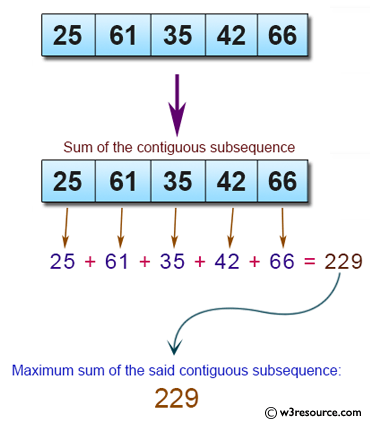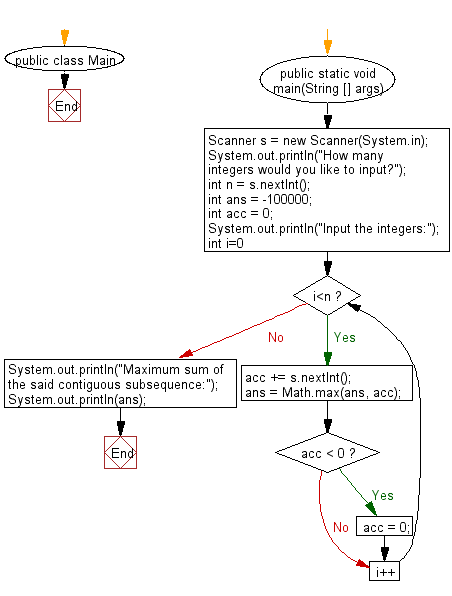# Java Exercises: Find the maximum sum of a contiguous subsequence from a given sequence of numbers

## Java Basic: Exercise-223 with Solution

Write a Java program to find the maximum sum of a contiguous subsequence from a given sequence of numbers a1, a2, a3, ... an. A subsequence of one element is also a continuous subsequence.

Input:
You can assume that 1 ≤ n ≤ 5000 and -100000 ≤ ai ≤ 100000.
Input numbers are separated by a space.
Input 0 to exit.

Pictorial Presentation:Sample Solution:

Java Code:

`````` import java.util.*;
public class Main {
public static void main(String [] args) {
Scanner s = new Scanner(System.in);
System.out.println("How many integers would you like to input?");
int n = s.nextInt();
int ans = -100000;
int acc = 0;
System.out.println("Input the integers:");
for (int i=0;i<n;i++) {
acc += s.nextInt();
ans = Math.max(ans, acc);
if (acc < 0) acc = 0;
}
System.out.println("Maximum sum of the said contiguous subsequence:");
System.out.println(ans);
}
}
```
```

Sample Output:

```How many integers would you like to input?
5
Input the integers:
25 61 35 42 66
Maximum sum of the said contiguous subsequence:
229
```

Flowchart:Java Code Editor:

What is the difficulty level of this exercise?

﻿

## Java: Tips of the Day

Array vs ArrayLists:

The main difference between these two is that an Array is of fixed size so once you have created an Array you cannot change it but the ArrayList is not of fixed size. You can create instances of ArrayLists without specifying its size. So if you create such instances of an ArrayList without specifying its size Java will create an instance of an ArrayList of default size.

Once an ArrayList is full it re-sizes itself. In fact, an ArrayList is internally supported by an array. So when an ArrayList is resized it will slow down its performance a bit as the contents of the old Array must be copied to a new Array.

At the same time, it's compulsory to specify the size of an Array directly or indirectly while creating it. And also Arrays can store both primitives and objects while ArrayLists only can store objects.

Ref: https://bit.ly/3o8L2KH